# A flow nozzle equipped with a differential pressure gage is used to measure the flow rate of water at 10°C (ρ 5= 999.7 kg/m3 and μ = 1.307 × 10–3 kg/m·s) through a 3-cm-diameter horizontal pipe. The nozzle exit diameter is 1.5 cm, and the measured pressure drop is 3 kPa. Determine the volume flow rate of water, the average velocity through the pipe, and the head loss. FIGURE P8–116

Question-AnswerCategory: Fluid MechanicsA flow nozzle equipped with a differential pressure gage is used to measure the flow rate of water at 10°C (ρ 5= 999.7 kg/m3 and μ = 1.307 × 10–3 kg/m·s) through a 3-cm-diameter horizontal pipe. The nozzle exit diameter is 1.5 cm, and the measured pressure drop is 3 kPa. Determine the volume flow rate of water, the average velocity through the pipe, and the head loss. FIGURE P8–116

A flow nozzle equipped with a differential pressure gage is used to measure the flow rate of water at 10°C (ρ 5= 999.7 kg/m3 and μ = 1.307 × 10–3 kg/m·s) through a 3-cm-diameter horizontal pipe. The nozzle exit diameter is 1.5 cm, and the measured pressure drop is 3 kPa. Determine the volume flow rate of water, the average velocity through the pipe, and the head loss.
FIGURE P8–116Step: 1

Calculate area of the pipe.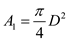Here, D is the diameter of the pipe.
Substitute 0.03 m for D.Calculate the area of the nozzle.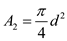Here, d is the diameter of the nozzle.
Substitute 0.015 m for d.Step: 2

Calculate the diameter ratio.Substitute 0.015 m for d and 0.03 m for D.Assume the flow is assumed to be steady and incompressible. And the coefficient of discharge for flow nozzle is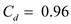.
Calculate the volume flow rate.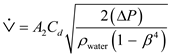…… (1)
Here,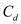is the coefficient of discharge,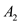is the area of the nozzle,is the pressure difference,is the diameter ratio,is the density of water.

Step: 3

Substitute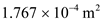forfor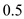forfor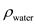and 3000 Pa forin equation (1).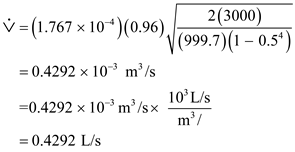.
Therefore, the volume flow rate of water,is.

Step: 4

Calculate the average velocity through the pipe.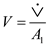Substitute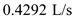forandfor.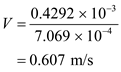Therefore, the average velocity through the pipe,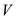is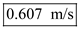.

Step: 5

Calculate the head loss through the nozzle.
Consider the Bernoulli’s theorem,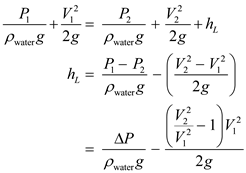…… (2)
Here,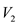is the final velocity,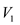is the initial velocity, g is the acceleration due to gravity.
Consider the relation of continuity equation,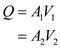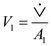…… (3)…… (4)

Step: 6

Calculate the initial velocity.

Consider equation (3),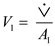Here,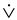is the volume flow rate.
Substituteforand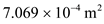for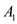.Calculate the ratio of velocities.
Consider equation (3), equation (4).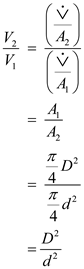…… (5)

Step: 7

Substitute 0.015 m for d and 0.03 m for D in equation (5).Calculate the head loss thorough the nozzle.
Substitutefor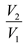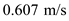for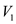for g,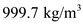forand 3000 Pa forin equation (2).Therefore, the head loss caused by nozzle meter,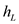is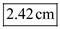.